Download Presentation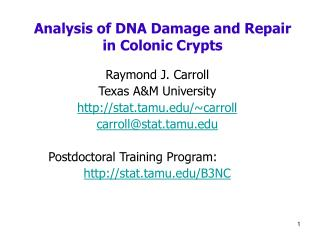Analysis of DNA Damage and Repair in Colonic Crypts

# Analysis of DNA Damage and Repair in Colonic Crypts - PowerPoint PPT Presentation

Analysis of DNA Damage and Repair in Colonic Crypts. Raymond J. Carroll Texas A&M University http://stat.tamu.edu/~carroll carroll@stat.tamu.edu Postdoctoral Training Program: http://stat.tamu.edu/B3NC. Acknowledgments. Jeffrey Morris , M.D. Anderson Lead authorI am the owner, or an agent authorized to act on behalf of the owner, of the copyrighted work described.
Download Presentation## Analysis of DNA Damage and Repair in Colonic Crypts

An Image/Link below is provided (as is) to download presentation

Download Policy: Content on the Website is provided to you AS IS for your information and personal use and may not be sold / licensed / shared on other websites without getting consent from its author.While downloading, if for some reason you are not able to download a presentation, the publisher may have deleted the file from their server.

- - - - - - - - - - - - - - - - - - - - - - - - - - E N D - - - - - - - - - - - - - - - - - - - - - - - - - -
Presentation Transcript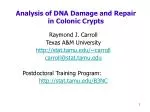### Analysis of DNA Damage and Repair in Colonic Crypts

Raymond J. Carroll

Texas A&M University

http://stat.tamu.edu/~carroll

carroll@stat.tamu.edu

Postdoctoral Training Program:

http://stat.tamu.edu/B3NC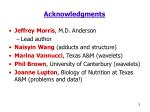Acknowledgments
• Jeffrey Morris, M.D. Anderson
• Naisyin Wang (adducts and structure)
• Marina Vannucci, Texas A&M (wavelets)
• Phil Brown, University of Canterbury (wavelets)
• Joanne Lupton, Biology of Nutrition at Texas A&M (problems and data!)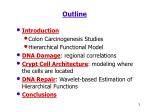Outline
• Introduction
• Colon Carcinogenesis Studies
• Hierarchical Functional Model
• DNA Damage: regional correlations
• Crypt Cell Architecture: modeling where the cells are located
• DNA Repair: Wavelet-based Estimation of Hierarchical Functions
• Conclusions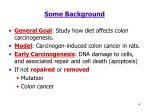Some Background
• General Goal: Study how diet affects colon carcinogenesis.
• Model: Carcinogen-induced colon cancer in rats.
• Early Carcinogenesis: DNA damage to cells, and associated repair and cell death (apoptosis)
• If not repaired or removed
• Mutation
• Colon cancer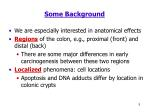Some Background
• We are especially interested in anatomical effects
• Regions of the colon, e.g., proximal (front) and distal (back)
• There are some major differences in early carcinogenesis between these two regions
• Localized phenomena: cell locations
• Apoptosis and DNA adducts differ by location in colonic crypts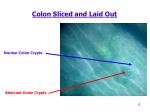Colon Sliced and Laid Out

Normal Colon Crypts

Aberrant Colon Crypts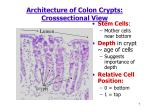Architecture of Colon Crypts: Crosssectional View
• Stem Cells:
• Mother cells near bottom
• Depth in crypt ~ age of cells
• Suggests importance of depth
• Relative Cell Position:
• 0 = bottom
• 1 = top

Lumen

crypts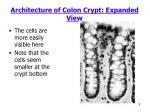Architecture of Colon Crypt: Expanded View
• The cells are more easily visible here
• Note that the cells seem smaller at the crypt bottom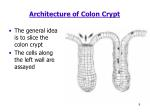Architecture of Colon Crypt
• The general idea is to slice the colon crypt
• The cells along the left wall are assayed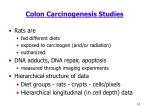Colon Carcinogenesis Studies
• Rats are
• fed different diets
• exposed to carcinogen (and/or radiation)
• euthanized.
• DNA adducts, DNA repair, apoptosis
• measured through imaging experiments
• Hierarchical structure of data
• Diet groups - rats - crypts - cells/pixels
• Hierarchical longitudinal (in cell depth) dataCoordinated Response
• Rats were exposed to a potent carcinogen (AOM)
• At both the proximal and distal regions of the colon, ~20 crypts were assayed
• The rat-level function is gdr(t)
• For each cell within each crypt, the level of DNA damage was assessed by measuring the DNA adduct levels
• Question: how is DNA damage related in the proximal and distal regions, across rats?
• We call this coordinated responseCoordinated Response as Correlation
• We are interested in the “correlation” of the DNA damage in the proximal region with that of the distal region
• Are different regions of the colon responding (effectively) independently to carcinogen exposure?
• This sort of interrelationship of response is what is being studied in our group.
• It is not cell signaling in the classic sense
• We will have data on this in the near futureCoordinated Response
• Correlation in the usual sense is not possible
• Let Y(t) = DNA adduct in a proximal cell measured by immunohistochemical staining intensity at cell depth t
• Let Z (t) = DNA adduct in a distal cell at cell depth t
• We cannot calculate correlation(Y,Z) (t) in the usual way
• the same cell cannot be in both locations
• Coordinated response then has to be measured at a higher levelCoordinated Response: Hierarchical Functional Model
• Let d = diet group
• Let r = rat
• Let c = crypt
• Let t =tdrc= cell position
• Let Ydrc(t) = adduct level in the proximal region
• The diet-level function is gd(t)
• Our aim: estimate the correlation between proximal and distal regions as a function of cell depth at the rat levelCoordinated Response: Average then Smooth
• If cell depths were identical for each crypt, we could solve this by “average then smooth”
• That is, average over all crypts at any given depth, then estimate the correlation as a function of depth
• The estimated correlation would of course account for the averaging over a finite number of crypts
• Problem: data are not of this structure
• Cell locations vary from crypt to crypt
• Number of cells varies from crypt-to-cryptCoordinated Response: Smooth then Average
• Instead, we smoothed crypts via nonparametric regression
• Then average the smooth fits over the crypts (on a grid of depths)
• Then compute the correlation as before
• We actually fit REML to the fitted functions at the crypt level
• Problem: Is there any effect due to the initial smooth?Coordinated Response: Asymptotics
• General theory available: kernel regression
• Allows explicit calculations
• Can we estimate the correlation function just as well as if the crypt-level functions were known?
• Complex higher order expansions necessary
• The asymptotic theory is for large numbers of
• Rats
• Crypts
• CellsCoordinated Response: Asymptotics
• Possibility #1: Use standard methods at the crypt level
• Optimal at the crypt level
• Double-smoothing phenomenon (at crypt then across crypts)
• Effect of smoothing does not disappearCoordinated Response: Asymptotics
• Possibility #2: Under-smoothing at crypt level
• Known to work for other double-smoothing problems
• Is optimal for this problem
• Explicit simple adjustments for under-smoothing derived
• Divide optimal bandwidth by the 1/5th power of the number of crypts
• Result: no asymptotic effect due to the initial smoothingCoordinated Response: Results
• Simulations: we found that this simple bit of under-smoothing works well.
• Data: extraordinary lack of sensitivity to the smoothing parameter
• other smoothers give the same basic answers
• In principle:
• Regular Smooth then Average: sub-optimal
• Undersmooth then Average: betterCoordinated Response: Asymptotics
• Alternatives:
• Random coefficient polynomial models: REML/Bayes
• Hierarchical regression splines
• Major Point:
• The method should not matter too much
• Estimation of Crypt level functions has no asymptotic effectThe negative correlation in the corn oil diet is unexpected

May suggest localization of damage: consistent with damage in the proximal or distal regions, but not bothFor basic reasons, as well as robustness reasons, we were led to study whether this was an artifact of the use of relative as opposed to actual cell depthModeling Cell Crypt Architecture
• Most analyses of cell depth measure cells on a relative basis
• Thus, if there are 11 cells, the depths are listed as 0/10, 1/10, …, 10/10
• This is not the same as actual depth
• Indeed, it effectively suggests that cells are uniformly spaced along the crypt wallCell Crypt Architecture: Two Questions
• We are interested in the first place in the architecture:
• Are the cells uniformly distributed within a crypt?
• It is also extremely tedious to measure actual cell depth
• Almost any statistical analysis extant uses nominal cell depth: i.e., cell i of n has nominal depth (i-1)/(n-1)
• Are downstream analyses affected by the use of nominal instead of actual cell depth?Cell Crypt Architecture: Two Questions
• Downstream analyses: affected by the use of nominal instead of actual cell depth?
• Let X = true cell depth = Beta(0.5,1.0) with n = 30
• Let W = nominal cell depth
• Let E(Y|X) = X
• What is E(Y|W)?
• Plot order statistics of X versus WCell Crypt Architecture
• We have data on 30 rats
• ~20 colonic crypts per rat
• ~45 cells per crypt
• For each rat, 3 crypts were analyzed to measure their actual cell positions
• Thus, we have incomplete data: true cell positions are missing on ~ 17 crypts per rat
• Question: is the negative proximal-distal correlation in the corn-oil group a consequence of measuring only nominal cell position?Cell Crypt Architecture: Order Statistics
• The actual cell positions are on [0,1]
• We model the true cell positions for each crypt as the order statistics from Beta(a,b)
• We fit the crypt level functions via parametric cubic random effects models
• General problem: data missing as a group but subject to ordering constraints
• The order statistic model greatly speeds up computationCell Crypt Architecture
• MCMC approach: various tricks to speed up especially the generation of the missing cell positions (~600 per animal)
• Missing cell positions can be generated simultaneously at the crypt level
• Simpler than cell-by-cell generation
• Faster than cell-by-cell generation
• If generation were cell-by-cell, the order constraints would have to be accounted forCell Crypt Architecture: Results
• Proximal architecture is almost exactly U[0,1]
• Distal architecture is clearly not uniform: Beta(a = 0.8,b = 1.0)
• Here is the posterior mean density
• The correlation analysis was virtually unchanged
• Appears that measuring exact cell positions is not necessaryCell DNA Damage and Repair
• The same data structure occurs for DNA repair enzyme data as it does for DNA damage (adduct) data
• It is clearly of great interest to understand the relationship between the two
• also as a function of cell depth
• Repair is measured on a pixel-by-pixel basis averaging across the crypt
• A problem arises: the DNA repair data are not nearly so smooth as the adduct dataDNA Repair Data Plots

DNA Repair Enzyme for Selected CryptsCell DNA Repair
• The irregularity of the DNA repair data suggests that new techniques are necessary
• We are going to use wavelet methods around an MCMC calculator
• The multi-level hierarchical data structure makes this a new problem
• The images are pixel-by-pixel:
• We “connected the dots”
• Split into 256 (2**8) “observations”
• Forces regularly spaced dataWavelets & Wavelet Regression
• Data space model: y = f(t) + e
• t = equally spaced grid, length n=2J, on (0,1)
• Heree = MVN(0,s2)
• In wavelet space: d = Wy =  + e*
• d = ‘empirical’ wavelet coefficients
•  = ‘true’ wavelet coefficients
• By orthogonality, e* ~ MVN(0,2)Overview of Wavelet Method
• Convert data Yabc to wavelet space dabc
• Involves 1 DWT for each crypt
• Fit hierarchical model in wavelet space to obtain
• Posterior distribution of ‘true’ wavelet coefficients dcorresponding to gd(t)
• Variance component estimates to assess relative variability
• Use IDWT to obtain posterior distribution of gd(t) for estimation and inferenceWavelet Space Model
• Wavelets: families of orthonormal basis functions
• ddrc = { } = Wydrc

Daubechies Basis Function

Discrete Wavelet TransformShrinkage Prior
• Prior on is a 0-normal mixture
• Nonlinear shrinkage -- denoises data
• regularization parameters
• Hierarchical model fit using MCMC• We focused on marginal (diet level) analyses
• The marginalization allowed for efficient MCMC
• Some fairly difficult calculations are required
• Much more efficient than brute-force
• Enables analysis of subsampling units, e.g., individual rats
• This we have not yet done in our data
• Enables assessment of variance componentsSummary
• Method to fit hierarchical longitudinal data
• Nonparametrically estimate mean profiles for:
• Treatments
• Individuals
• Subsampling units
• Estimates of relative variability at hierarchical levels
• We find that 90% of the variability is from crypt-to-crypt
• Do lots of crypts!0 h

12 h

3 h

6 h

9 h

Fish Oil

Corn OilConclusion
• Cell-based colon carcinogenesis studies
• Hierarchical Longitudinal/Functional data
• Rich in information -- challenging to extract
• Methods developed
• Kernel methods for longitudinal correlations
• Method for missing data with order constraints
• Wavelet regression methods for longitudinal hierarchical data